### Home > CAAC > Chapter 12 > Lesson 12.1.2 > Problem12-21

12-21.
1. If f(x) = 3x − 9 and g(x) = −x2, find: Homework Help ✎

1. f(−2)

2. g(−2)

3. x if f(x) = 0

4. g(m)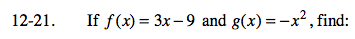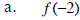Substitute the value for x.
f(−2) = 3(−2) − 9
Continue to simplify.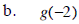Substitute the value for x.
g(−2) = −(−2)2
Continue to simplify.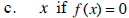Substitute 0 for f(x).
0 = 3x −9
Continue solving for x.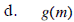Substitute the value for x.
g(m) = −m2